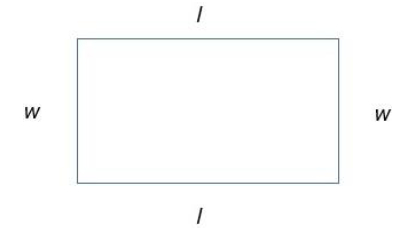# Java Program to Find the Perimeter of a Rectangle

In this article, we will understand how to find the perimeter of a rectangle. The Perimeter of a rectangle is calculated by adding the lengths of all the sides of the rectangle.

Below is a demonstration of a rectangle. The perimeter of a rectangle is the total length of the two lengths and two widths of the rectangle −Input

Suppose our input is −

The length of the sides of a rectangle are : 5, 8, 5, 8

Output

The desired output would be −

Perimeter : 26


## Algorithm

Step 1 – START
Step 2 – Declare 5 floating point variables a, b, c, d and perimeter
Step 3 – Read values of a and b from user as the opposite sides of the rectangle are equal,
only two sides input will be sufficient.
Step 4- Calculate the perimeter by adding up all the sides
Step 5- Display the Perimeter value
Step 6 – STOP

## Example 1

Here, the input is being entered by the user based on a prompt. You can try this example live in ourcoding ground tool.

import java.util.Scanner;
public class RectanglePerimeter{
public static void main (String args[]){
float a ,b, c, d, perimeter;
System.out.println("Required packages have been imported");
Scanner my_scanner = new Scanner(System.in);
System.out.println("A Scanner object has been defined ");
System.out.print("Enter the length of first side : ");
a = my_scanner.nextFloat();
System.out.print("Enter the length of second side : ");
b = my_scanner.nextFloat();
c = a;
d = b;
System.out.printf("The length of the sides of the Rectangle are %.2f %.2f %.2f %.2f", a, b, c, d);
perimeter = a + b + c + d;
System.out.printf("\nThe perimeter of Rectangle is: %.2f",perimeter);
}
}

## Output

Required packages have been imported
A Scanner object has been defined
Enter the length of first side : 5
Enter the length of second side : 8
The length of the sides of the Rectangle are 5.00 8.00 5.00 8.00
The perimeter of Rectangle is: 26.00

## Example 2

Here, the integer has been previously defined, and its value is accessed and displayed on the console.

public class RectanglePerimeter{
public static void main (String args[]){
float a ,b, c, d, perimeter;
a=c= 8;
b=d= 5;
System.out.printf("The length of the sides of the Rectangle are %.2f %.2f %.2f %.2f", a, b, c, d);
perimeter = a + b + c + d;
System.out.printf("\nThe perimeter of Rectangle is: %.2f",perimeter);
}
}

## Output

The length of the sides of the Rectangle are 8.00 5.00 8.00 5.00
The perimeter of Rectangle is: 26.00